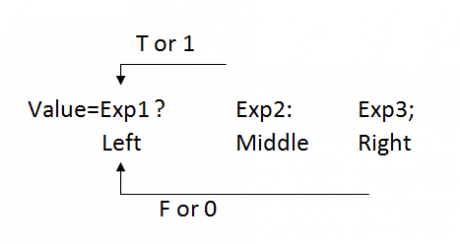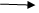Conditional operators in C
Ratings:
(4)
Views:909## Explain about Conditional operators in C Language with Examples

Conditional operators are terinary category operators.

• Terinary category means it requires 3 arguments i.e left, middle and right side arguments.
• In conditional operator, when expression is true, then returns with middle argument, if condition is false, then returns with right side argument and left side argument is treated as condition.

### Syntax:According to the syntax, when expression 1 is True or L value non zero , then exp2 or middle value returns.   If exp1 is false or “L” value is Zero, then exp 3 or right side value will returns

• When we are working with conditional operators. We require to satisfy following conditions.

i.e No of question marks and colons should be equalEvery colon should match with just before the “}” markEvery colon should followed by “}”mark only   Int a; A=10?20:30; M.R A=2>5!=1?10:20;               Expression is true collects middle data A=1?10:20;   10   A=2<5 && 578910:20; 1&&0 9 10:20; A=0 ? 10: 20; 20           false, returns right side value   A= 2>5 ? 10:20:30;       no.of colons and “?” markes should be same   A=5<8 ? 10:20:5!=5?30; Every colon should followed by ? mark. A=8>5!=1?10:5<2==0?20:30; upto 1st ? – left side value 0!=1?10: 90==0!20:30          after 1st question mark and Before first colon is middle value 0?10: 1?20:30 Remaining is right side value A=1!>1?10:/  5<2==0?20:30; L             M  R 0=0 A=20   A=2<5?   5>2!=0 10:20:30; L                     2   2      R 2<5- True returns middle so, consider 5>2!=0?10:20; 1!=0?10:20; 1? 10:20,10 1!=1   8 a=1!=2<5?10=15!=0?20:8<5!=0?30:40; L                   R 0?10: !5!=0?20:8<5!=0?30:40!5=false L           M    R 0?10:15!=0?20:8<5!=0?30:40   0!=0 8<5!=0 ? 30:40 L              M   R   9   a=2>5!=8<5?10=1!=5>8?!5?20:30:40; L                           R   1!=5>8?!5?20:30:40; L            M             R !=5?20:30;30 True 10 a=5<8?2>5!=1?5!=5==0?10:20:30:40;   2>5!=1!!5!=5==0?10:20:30; L !5!=5==0?10:20; L                 M  R 11 a=2>5?18=0?10:20:8>2!=0?5<8!=1?30:!5!=5?1?40:2>5!50:60:70:80   6>2!=0?5<8!=1?30:!5!=5?!1?40=2>5?50:60:70:80   5<8!=1?30:!5!=5?!1?40:2>5?50:60:70;   !5!=5?!1?40:2>5?50:60:70   !1! 40:2>5?50:60; 2>5?50=60; 60

• The basic advantage of conditional operator is it increase the execution speed of the program
• Generally conditional operators are used to reduce the coding part. When coding part is reducing, it occupies less memory. So, automatically performance will increase.

## Nested if else:

When we are placing the if condition within the condition. Then it is called Nested if NIf can be applied upto 255 blocks. When we are working with nested if else at even any point of time only block will be executed. Syntax :- If(condition1) { Block1; } Else { If (condition 2) { Block2; } Else { If (condition 3) Block 3; Else Block4; } } Acc to the syntax if condition 1 is true then blocks will executed. If it false then control will pass through else port within the else part if condition 2 is true, then block 2 will executed if it is false then control will pass to nested else. Within the nested else if condition 3 is true then block 3 is executed else block 4 will executed. Void main() { Int x, y, z, min ; X=10;y=5;z=20; If(x<y && x<z) { Min=y; } If(z<x && Z<Y) { Min=z } Printf(“min value:%d”,min); } o/p : min value=5

• In implementation when we are constructing inter related conditions independently after completion of requirement compiler will check rest of conditions even through if it is false.
• Whenever we having inter related conditions, then always recommended to create optional block by using else port.
• According to the syntax if condition 1 is true then block will executed ,if it is false then control will pass through else port within the else part if condition 2 is true, then block2 will executed if it is false then control will pass to nested else.
• Within the nested else if condition 2 is true and then block3 is executed else block 4 will executed.

Void main() { Int x,y,z, min; X=10,y=5,z=20; If (x<y&&y<z) { Min=y; } If (z<x&&z<y) { Min=y; } If(z<x&&z<y) { Min=z; } Printf (“min value =%d”,min); } O/P : min value =5

• In implementation when we are constructing inter related conditions independently after completion of requirement complier will check rest of conditions eve though if it is false.
• Whenever we having inter related conditions, then always recommended to create optional block by using else part.
• When we having only one alternate block then go for else part, if we having multiple alternate blocks then recommended to go for nested else.
• In above prog, in place of constructing multiple conditions we can create a single statement which can provide min value i.e condition operator are required to use.
• Void main()

{ Int x,y,z ,min; X=10;y=5;z=20; Min=x<y && x<Z?x:y<Z?y:Z; Printf(“min value:%d”,min); } o/p:  min value=5         Void main() { Int a,b,c,d,max; Clrscr(); Printf(“Enter values”) Scanf(“%d%d%d%”,&a,&b,&c,&d); //a=100,b=50,c=20,d=30; If(a>b && a>c && a>d) { Max=a; } Else { If(b>c && b>d) { Max=b; } Else { If(c>d) } Printf(“max value=%d”,max); Getch(); }   o/p : Enter 4 values : 10 20 50 30 max value = 50     By using conditional operator   Void main() { Int a,b,c,d,max; Clrscr(); Printf("Enter 4 values :”); Scanf(“%d %d %d %d”,&a,&b,&c,&d); Max=a>b && a>c && a>d ? a:b>c && b>d?b:c>d?ad; Printf(“Max value=%d”,max); Getch(); } o/p : enter 4 values =50

## By using conditional operator

Void main() { Int a,b,c,d,max; Clrscr(); Printf("Enter 4 values :”); Scanf(“%d %d %d %d”,&a,&b,&c,&d); Max=a>b && a>c && a>d ? a:b>c && b>d?b:c>d?ad; Printf(“Max value=%d”,max); Getch(); } o/p : enter 4 values =50

## Electricity Bill program:-

Void main() { Int sno, cread, pread, nunits,t; Float rps; Clrscr(); Printf(“Enter sno:”); Scanf(“%d”,&sno); If(sno==4235) { Printf(”\Enter current reading :”); Scanf(“%d”,& cread); Nunits=cread-pread; If(nunits>=151) { T=nunits-150; Rps=t*6.0;                              // 4rth Rps=rps+50+5.0;          // 4+3rd Rps=rps+50*3.50;                  // 4+3rd+2nd Rps=rps+50*2.0;          // 4+3+2+1st } Else if (nunits>=101 && nunits <=150) { T=nunits-100; Rps=t*5.0;                    // 3rd Rps=rps+50*3.50;        // 3+2nd Rps=rps+50*2.0; // 3+2+1st } Else if(nutits>=51 && nunits<=100) { T=nunits-50; Rps=t*3.50; Rps=rps+50*2.0; } Else Rps=nunits * 2.0; If(rps<64) Ops=64.0;                   // min amount Printf(“\n total amount value:%f”,rps); } Else Printf(“\n invalid sno”); Getch(); }     Execution: Enter SNo: 1234 Invalid sno Enter SNo:4235 Enter current reading : 180 Total amount value : 105.00 In printf f’n , when we are passing “ percentage.2f ” format specifiers then fractional part it displays only two digits.